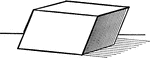### Parallelopiped

Illustration of a parallelopiped - a prism with a parallelogram as its base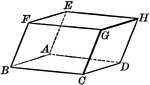### Parallelopiped

Illustration of a parallelopiped - a prism with a parallelogram as its base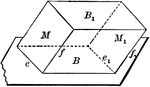### Equal and Parallel Opposite Faces of a Parallelopiped

Diagram used to prove the theorem: "The opposite faces of a parallelopiped are equal and parallel."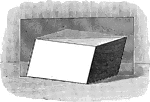### Parallelopiped with Plane Passing Through

Illustration of a parallelopiped with a plane passing through two diagonally opposite edges. The plane…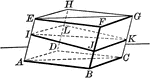### Parallelopiped with Plane Passing Through

Illustration of a parallelopiped with a plane passing through two diagonally opposite edges. The plane…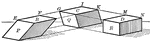### Parallelopiped Showing Volume

Illustration of a parallelopiped (a prism with a parallelogram as its base) used to demonstrate that…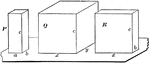### Relationship Between 2 Parallelopipeds With Equal Altitudes

Diagram used to prove the theorem: "The rectangular parallelopipeds which have two dimensions in common…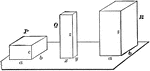### Relationship Between Dimensions of Parallelopipeds

Diagram used to prove the theorem: "The rectangular parallelopipeds are to each other as the product…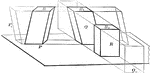### Volume of Parallelopiped

Diagram used to prove the theorem: "The volume of a any parallelopiped is equal to the product of its…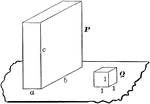### Volume of Rectangular Parallelopiped

Diagram used to prove the theorem: "The volume of a rectangular parallelopiped is equal to the product…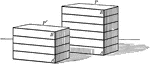### Rectangular prisms

Illustration of 2 parallelopipeds/rectangular prisms.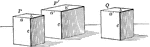### Rectangular prisms

Illustration of 3 parallelopipeds/rectangular prisms.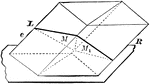### Parallelopiped Divided Into Triangular Prisms

Diagram used to prove the theorem: "The plane passed through two diagonally opposite edges of a parallelopiped…